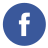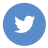### Java Loop Control

java

In Java, loop control statements are used to execute a block of code multiple times. There are three types of loop control statements in Java: for, while, and do-while.

1. for loops are used to execute a block of code a specific number of times. The syntax for a for loop is as follows:
``````for (initialization; condition; increment/decrement) {
// code to be executed
}``````

The initialization expression is executed once before the loop starts. It is usually used to initialize a loop counter. The condition is evaluated before each iteration of the loop. If the condition is true, the loop body is executed. If the condition is false, the loop is terminated. The increment/decrement expression is executed after each iteration of the loop. It is usually used to update the loop counter.

Here is an example of a for loop that prints the numbers from 1 to 10:

``````for (int i = 1; i <= 10; i++) {
System.out.println(i);
}``````
1. while loops are used to execute a block of code repeatedly as long as a condition is true. The syntax for a while loop is as follows:
``````while (condition) {
// code to be executed
}``````

The condition is evaluated before each iteration of the loop. If the condition is true, the loop body is executed. If the condition is false, the loop is terminated.

Here is an example of a while loop that prints the numbers from 1 to 10:

``````int i = 1;
while (i <= 10) {
System.out.println(i);
i++;
}``````
1. do-while loops are similar to while loops, but the loop body is executed at least once before the condition is evaluated. The syntax for a do-while loop is as follows:
``````do {
// code to be executed
} while (condition);``````

The loop body is executed first, and then the condition is evaluated. If the condition is true, the loop body is executed again. If the condition is false, the loop is terminated.

Here is an example of a do-while loop that prints the numbers from 1 to 10:

``````int i = 1;
do {
System.out.println(i);
i++;
} while (i <= 10);``````